# A modest STL tutorial

I am using a software tool called hyperlatex to create this document. The tutorial is also available in gzip-compressed postscript form or zip-compressed postscript form. For those wanting to install a copy of these pages on their system, they may be freely copied providing they are not modified in any significant way (other than, say, locale changes). The file tut.tar.Z contains a tarred distribution of the pages. Please note that I will be making modifications to this document in the coming months, so you may want to occasionally check for changes. I will start putting in version numbers, and if I can manage to, changebars.

## Disclaimer

I started looking at STL in 1995; for a long time I could compile only minimal subsets of the HP version of the library. More recently, the compilers used by the students I teach have been able to support more of STL, and I have been adding it to the introductory and advanced courses I teach. Now, at the beginning of 1998, I use STL in the labs for all of the courses I teach.

I have been using C++ since 1988, and teaching C++ and object-oriented design courses in industry since 1990. I really like the design philosophies in STL; I think that you can learn a great deal about how generalization can simplify programming by understanding why STL is constructed the way it is.

Mark Nelson's book on STL is very good if you want to understand the internal details, but is probably overkill for many people. Musser and Saini have a good book on STL, but it is a bit out-of-date.

I haven't seen very much online documentation on STL, apart from the good but rather dense paper by Stepanov and Lee, I thought I would try to write something to give people a taste of what a good library will be do for them.

Another reason for getting people to start trying out STL soon is to put pressure on the compiler-writers to get their compilers patched up enough to take the strain it puts on them...

I would greatly appreciate comments or suggestions from anyone.

## Outline

STL contains five kinds of components: containers, iterators, algorithms, function objects and allocators.

In the section Example I present a simple example, introducing each of the five categories of STL components one at a time.

In the section Philosophy I explain the rationale behind the organization of STL, and give some hints on the best ways to use it. (Not yet written)

The Components section goes into each category of component in more detail.

The section Extending STL shows how to define your own types to satisfy the STL requirements. (Not yet written)

## A first example

Most of you probably use some kind of auto-allocating array-like type. STL has one called `vector`. To illustrate how vector works, we will start with a simple C++ program that reads integers, sorts them, and prints them out. We will gradually replace bits of this program with STL calls.

### Version 1: Standard C++

Here is a standard C++ program to read a list of integers, sort them and print them:
```#include <stdlib.h>
#include <iostream.h>

// a and b point to integers.  cmp returns -1 if a is less than b,
// 0 if they are equal, and 1 if a is greater than b.
inline int cmp (const void *a, const void *b)
{
int aa = *(int *)a;
int bb = *(int *)b;
return (aa < bb) ? -1 : (aa > bb) ? 1 : 0;
}

// Read a list of integers from stdin
// Sort (c library qsort)
// Print the list

main (int argc, char *argv[])
{
const int size = 1000;  // array of 1000 integers
int array [size];
int n = 0;
// read an integer into the n+1 th element of array
while (cin >> array[n++]);
n--; // it got incremented once too many times

qsort (array, n, sizeof(int), cmp);

for (int i = 0; i < n; i++)
cout << array[i] << "\n";
}
```

### Version 2: containers, iterators, algorithms

STL provides a number of container types, representing objects that contain other objects. One of these containers is a class called `vector` that behaves like an array, but can grow itself as necessary. One of the operations on `vector` is `push_back`, which pushes an element onto the end of the vector (growing it by one).

A vector contains a block of contiguous initialized elements -- if element index k has been initialized, then so have all the ones with indices less than k.

A vector can be presized, supplying the size at construction, and you can ask a vector how many elements it has with `size`. This is the logical number of elements -- the number of elements up to the highest-indexed one you have used. There is a also a notion of capacity -- the number of elements the vector can hold before reallocating.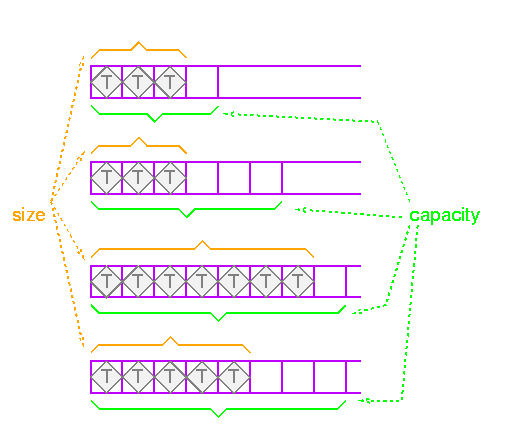Let's read the elements and push them onto the end of a vector. This removes the arbitrary limit on the number of elements that can be read.

Also, instead of using qsort, we will use the STL sort routine, one of the many algorithms provided by STL. The sort routine is generic, in that it will work on many different types of containers. The way this is done is by having algorithms deal not with containers directly, but with iterators.

#### Preview of iterators

I'll go into iterators in detail later, but for now here is enough to get started.

Iterators provide a way of specifying a position in a container. An iterator can be incremented or dereferenced, and two iterators can be compared. There is a special iterator value called "past-the-end".

You can ask a vector for an iterator that points to the first element with the message `begin`. You can get a past-the-end iterator with the message `end`. The code

```vector<int> v;
// add some integers to v
vector::iterator i1 = v.begin();
vector::iterator i2 = v.end();
```
will create two iterators like this: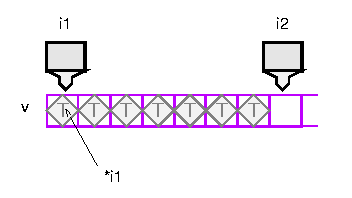Operations like sort take two iterators to specify the source range. To get the source elements, they increment and dereference the first iterator until it is equal to the second iterator. Note that this is a semi-open range: it includes the start but not the end.

Two vector iterators compare equal if they refer to the same element of the same vector.

Putting this together, here is the new program:

```#include <string.h>
#include <algo.h>
#include <vector.h>
#include <stdlib.h>
#include <iostream.h>

main ()
{
vector<int> v;  // create an empty vector of integers
int input;
while (cin >> input)    // while not end of file
v.push_back (input);  // append to vector

// sort takes two random iterators, and sorts the elements between
// them.  As is always the case in STL, this includes the value
// referred to by first but not the one referred to by last; indeed,
// this is often the past-the-end value, and is therefore not
// dereferenceable.
sort(v.begin(), v.end());

int n = v.size();
for (int i = 0; i < n; i++)
cout << v[i] << "\n";
}
```
Incidentally, this is much faster than qsort; at least a factor of 20 on the examples I tried. This is presumably due to the fact that comparisons are done inline.

In addition to iterating through containers, iterators can iterate over streams, either to read elements or to write them.

An input stream like `cin` has the right functionality for an input iterator: it provides access to a sequence of elements. The trouble is, it has the wrong interface for an iterator: operations that use iterators expect to be able to increment them and dereference them.

STL provides adaptors, types that transform the interface of other types. This is very much how electrical adaptors work. One very useful adaptor is `istream_iterator`. This is a template type (of course!); you parameterize it by the type of object you want to read from the stream. In this case we want integers, so we would use an `istream_iterator<int>`. Istream iterators are initialized by giving them a stream, and thereafter, dereferencing the iterator reads an element from the stream, and incrementing the iterator has no effect. An istream iterator that is created with the default constructor has the past-the-end value, as does an iterator whose stream has reached the end of file.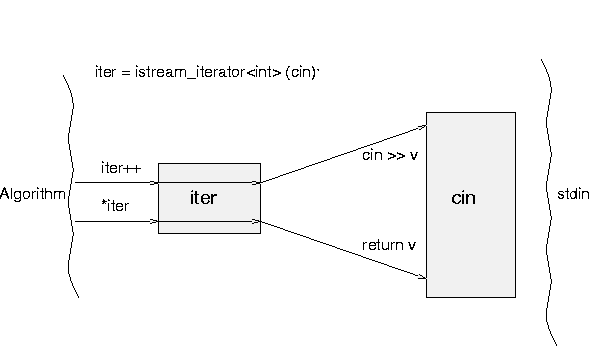In order to read the elements into the vector from standard input, we will use the STL `copy` algorithm; this takes three iterators. The first two specify the source range, and the third specifies the destination.

The names can get pretty messy, so make good use of typedefs. Iterators are actually parameterized on two types; the second is a distance type, which I believe is really of use only on operating systems with multiple memory models. Here is a typedef for an iterator that will read from a stream of integers:

```typedef istream_iterator<int,ptrdiff_t> istream_iterator_int;
```
The second argument to the template should default to ptrdiff_t, but most compilers do not understand default template arguments. Some implementations of STL define `istream_iterators` with only one parameter, and supply a hard-coded distance type. So you will have to see whether your compiler understands default template arguments; if it does, you can declare the iterator type like this:
```typedef istream_iterator<int> istream_iterator_int;
```
So to copy from standard input into a vector, we can do this:
```copy (istream_iterator_int (cin), istream_iterator_int (), v.begin());
```
The first iterator will be incremented and read from until it is equal to the second iterator. The second iterator is just created with the default constructor; this gives it the past-the-end value. The first iterator will also have this value when the end of the stream is reached. Therefore the range specified by these two iterators is from the current position in the input stream to the end of the stream.

There is a bit of a problem with the third iterator, though: if v does not have enough space to hold all the elements, the iterator will run off the end, and we will dereference a past-the-end iterator (which will cause a segfault).

What we really want is an iterator that will do insertion rather than overwriting. There is an adaptor to do this, called `back_insert_iterator`. This type is parameterized by the container type you want to insert into.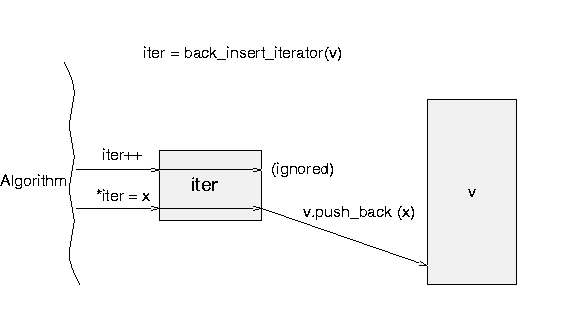So input is done like this:

```  typedef istream_iterator<int,ptrdiff_t> istream_iterator_int;

vector<int> v;
istream_iterator_int start (cin);
istream_iterator_int end;
back_insert_iterator<vector<int> > dest (v);

copy (start, end, dest);
```
Similarly, to print out the values after sorting, we use copy:
```  copy (v.begin(), v.end(), ostream_iterator<int> (cout, "\n"));
```
`ostream_iterator` is another adaptor; it provides output iterator functionality: assigning to the dereferenced iterator will write the data out. The `ostream_iterator` constructor takes two arguments: the stream to use and the separator. It prints the separator between elements.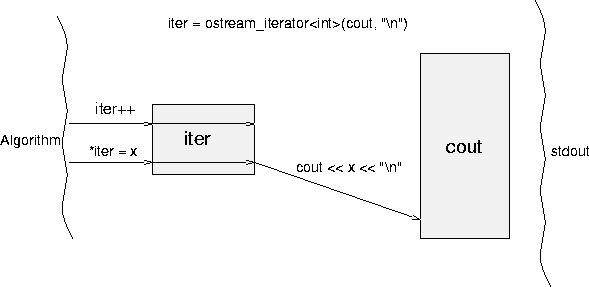Putting this all together,

```#include <string.h>
#include <algo.h>
#include <vector.h>
#include <stdlib.h>
#include <iostream.h>

main ()
{
vector<int> v;
istream_iterator<int,ptrdiff_t> start (cin);
istream_iterator<int,ptrdiff_t> end;
back_insert_iterator<vector<int> > dest (v);

copy (start, end, dest);
sort(v.begin(), v.end());
copy (v.begin(), v.end(), ostream_iterator<int>(cout, "\n"));
}
```

### Discussion

I find the final version of the program the cleanest, because it reflects the way I think of the computation happening: the vector is copied into memory, sorted, and copied out again.

In general, in STL, operations are done on containers as a whole, rather than iterating through the elements of the container explicitly in a loop. One obvious advantage of this is that it lends itself easily to parallelization or hairy optimizations (e.g., one could be clever about the order the elements were accessed in to help avoid thrashing).

Most of the STL algorithms apply to ranges of elements in a container, rather than to the container as a whole. While this is obviously more general than having operations always apply to the entire container, it makes for slightly clumsy syntax. Some implementations of STL (e.g., ObjectSpace), provide supplementary versions of the algorithms for common cases. For example, STL has an algorithm `count` that counts the number of times a particular element appears in a container:

```template <class InputIterator, class T, class Size>
void count (InputIterator start, InputIterator end, const T& value, Size& n);
```
To find how many elements have the value 42 in a vector `v`, you would write:
```int n = 0;
count (v.begin(), v.end(), 42, n);
```
ObjectSpace defines an algorithm `os_count` that provides a simpler interface:
```int n = os_count (v, 42);
```

## Philosophy

The Standard Template Library is designed for use with a style of programming called generic programming. The essential idea behind generic programming is to create components that can be composed easily without losing any performance. In some sense, it moves the effort that is done at run-time in object-oriented programming (dynamic binding) to compile-time, using templates.

## STL components

### Containers

Containers are objects that conceptually contain other objects. They use certain basic properties of the objects (ability to copy, etc.) but otherwise do not depend on the type of object they contain.

STL containers may contain pointers to objects, though in this case you will need to do a little extra work.

vectors, lists, deques, sets, multisets, maps, multimaps, queues, stacks, and priority queues, did I miss any? are all provided.

Perhaps more importantly, built-in containers (C arrays) and user-defined containers can also be used as STL containers; this is generally useful when applying operations to the containers, e.g., sorting a container. Using user-defined types as STL containers can be accomplished by satisfying the requirements listed in the STL container requirements definition.

If this is not feasible, you can define an adaptor class that changes the interface to satisfy the requirements.

All the types are "templated", of course, so you can have a vector of ints or Windows or a vector of vector of sets of multimaps of strings to Students. Sweat, compiler-writers, sweat!

To give you a brief idea of the containers that are available, here is the hierarchy: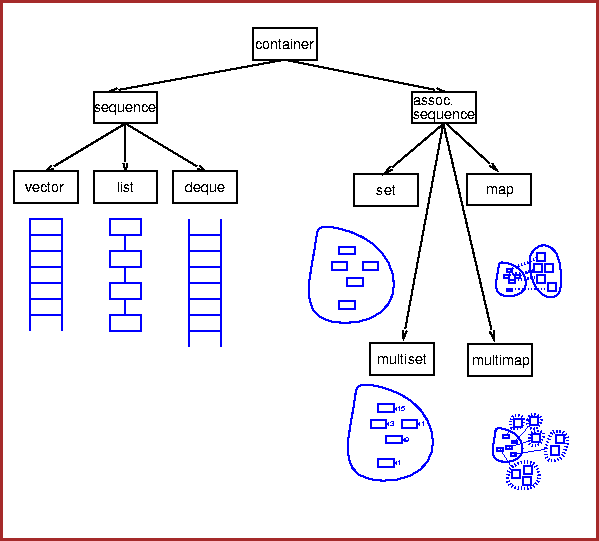#### Sequences

Contiguous blocks of objects; you can insert elements at any point in the sequence, but the performance will depend on the type of sequence and where you are inserting.

##### Vectors
Fast insertion at end, and allow random access.

##### Lists
Fast insertion anywhere, but provide only sequential access.
##### Deques
Fast insertion at either end, and allow random access. Restricted types, such as stack and queue, are built from these using adaptors.
##### Stacks and queues

Provide restricted versions of these types, in which some operations are not allowed.

#### Associative containers

Associative containers are a generalization of sequences. Sequences are indexed by integers; associative containers can be indexed by any type.

The most common type to use as a key is a string; you can have a set of strings, or a map from strings to employees, and so forth.

It is often useful to have other types as keys; for example, if I want to keep track of the names of all the Widgets in an application, I could use a map from Widgets to Strings.

##### Sets

Sets allow you to add and delete elements, query for membership, and iterate through the set.

##### Multisets

Multisets are just like sets, except that you can have several copies of the same element (these are often called bags).

##### Maps
Maps represent a mapping from one type (the key type) to another type (the value type). You can associate a value with a key, or find the value associated with a key, very efficiently; you can also iterate through all the keys.
##### Multimaps

Multimaps are just like maps except that a key can be associated with several values.

Should add other containers: priority queue, bit vector, queue.

### Examples using containers

Here is a program that generates a random permutation of the first `n` integers, where `n` is specified on the command line.

```#include <iostream.h>
#include <vector.h>
#include <algo.h>
#include <iterator.h>

main (int argc, char *argv[])
{
int n = atoi (argv); // argument checking removed for clarity

vector<int> v;
for (int i = 0; i < n; i++)            // append integers 0 to n-1 to v
v.push_back (i);

random_shuffle (v.begin(), v.end());                         // shuffle
copy (v.begin(), v.end(), ostream_iterator<int> (cout, "\n")); // print
}
```
This program creates an empty vector and fills it with the integers from 0 to `n`. It then shuffles the vector and prints it out.

It is quite common to want a sequence of elements with arithmetically increasing values; common enough that there is an algorithm that does something like this for us. It is called iota:

```template <class ForwardIterator, class T>
void iota (ForwardIterator first, ForwardIterator last, T value);
```
This function allows us to fill a range of a container with increasing values, starting with some initial value:
```vector<int> a(100); // initial size 100

iota (a.begin(), a.end(), 0);
```
This call will fill the array `a` with the values 0, 1, 2...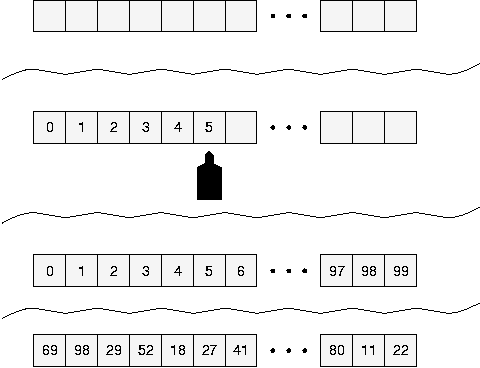Unfortunately, this is not quite what we wanted -- this overwrites an existing vector, whereas in our case, we had an empty vector, and we wanted the elements appended to it. There are two problems here. The first is that the termination condition for the `iota` function is specified by an iterator; the loop terminates when the moving iterator becomes equal to the terminal iterator.

Many algorithms in STL come in several flavors, corresponding to different terminating conditions. For example, `generate` uses two iterators to specify a range; `generate_n` uses one iterator and an integer to specify the range.

The `iota` function, unfortunately, does not have an `iota_n` counterpart, but it is very easy to write:

```template <class ForwardIterator, class T>
void iota_n (ForwardIterator first, int n, T value)
{
for (int i = 0; i < n; i++)
*first++ = value++;
}
```
In order to append to the vector instead of overwriting its contents, we will use an adaptor `back_inserter`:

```#include <iostream.h>
#include <vector.h>
#include <algo.h>
#include <iterator.h>

main (int argc, char *argv[])
{
int n = atoi (argv); // argument checking removed for clarity

vector<int> v;
iota_n (v.begin(), 100, back_inserter(v));

random_shuffle (v.begin(), v.end());                         // shuffle
copy (v.begin(), v.end(), ostream_iterator<int> (cout, "\n")); // print
}
```
`back_inserter` is a function that takes a container as an argument, and returns an iterator. The iterator is defined in such a way that writing a value through it and incrementing it will cause the value to be appended to the container.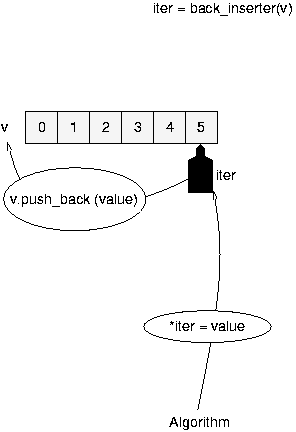### Iterators

Iterators are like location specifiers for containers or streams of data, in the same way that an `int*` can be used as a location specifier for an array of integers, or an ifstream can be used as a location specifier for a file. STL provides a variety of iterators for its different collection types and for streams.

#### Input iterators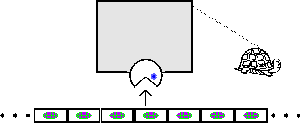Input iterators provide access to data sources. The source may be an STL container, another type of container, a stream, a virtual source (such as a set of permutations), etc.

#### Output iterators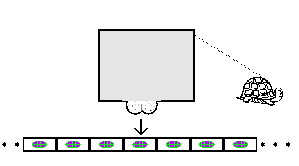Output iterators provide access to data sinks: locations to store the results of a computation. The sink may be an STL container, a user-defined container, a stream, etc.

#### Using input and output iterators

Just input and output iterators are enough to do quite a lot, since many operations boil down to copying objects around. For example, this function copies all the elements of a container `v` to standard output. `ostream_iterator` is an adaptor; it is an output iterator type. The iterator operations are defined so that in the case below, assigning through the iterator prints to standard output, with each print followed by a newline.

```copy (v.begin(), v.end(), ostream_iterator<int> (cout, "\\n"));
```
The first two arguments specify the source data: start an iterator that points to the beginning of the vector v, and keep going until the iterator compares equal with v.end(), which is called a past-the-end value. Almost all STL operations have one or more pairs of input iterators specifying the data to work with.

The third argument is an adaptor that turns an ostream like cout into an output iterator; don't worry about the details for now.

A similar operation reads data into a vector (for now, we will assume the vector has enough space allocated already):

```copy (istream_iterator<int> (cin), istream_iterator<int> (), v.begin());
```
Moreover, input and output iterators are necessary to do many things, since we usually need to specify what data we want to work with, and where to put the result.

#### Forward iterators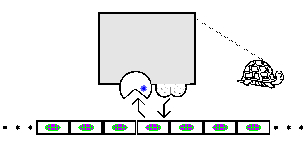A forward iterator allows traversal of the sequence, reading and/or writing each element, but no backing up. Many algorithms request a forward iterator, for example

```long *p = new int ;
fill (p, p+100, 0);
```
Fill's first two arguments specify the range the operation should take place on, and the third specifies the value to write through the iterator at each position.

#### Bidirectional iterators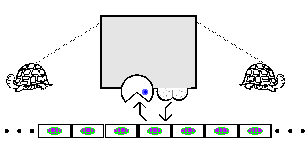Bidirectional iterators can be moved forward or backward, and can be used to read or write the elements of the sequence. Moving an iterator from one part of the sequence to another takes time proportional to the number of elements between the two.

#### Containers and iterators

All the STL containers provide at least bidirectional iterators: lists, sets, maps, and so on can be traversed forward or backward. Some containers provide even more flexible indexing:

#### Random access iterators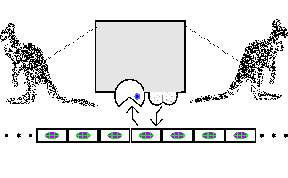Random access iterators can jump from any place to any other place in constant time I am not sure that this is required, and it is certainly allowed to be amortized constant time. Every C pointer type is an STL random iterator for a C array container. If you have a random-access container, you can perform all sorts of nifty operations on it, such as mapping over a vector, with or without accumulation, finding and replacing elements satisfying predicates, partitions, shuffling, and many more. The extent of this library never ceases to amaze me given that the source code is really quite small. I think that is a testimony to this kind of generic programming.

### Algorithms

The STL algorithms are template C++ functions to perform operations on containers. In order to be able to work with many different types of containers, the algorithms do not take containers as arguments. Instead, they take iterators that specify part or all of a container. In this way the algorithms can be used to work on entities that are not containers; for example, the function `copy` can be used to copy data from standard input into a vector.

Some algorithms require only the capabilities of input iterators, while others require random access (e.g., to sort).

The algorithms include sorting operations (sort, merge, min, max...), searching operations (find, count, equal...), mutating operations (transform, replace, fill, rotate, shuffle...), and generalized numeric operations (accumulate, adjacent difference...).

### Function objects

Function objects are STL's way of representing "executable data". For example, one of the STL algorithms is `for_each`. This applies a function to each object in a container. You need to be able to specify what to do to each object in the container.

#### Overview

Function objects are objects on which at least one parenthesis operation `(. . .)` is defined. They are used for three main purposes: generating data, testing data (predicates), and applying operations.

#### Generators

Algorithms like `generate` walk through a range, calling a function object at each step, and assigning the result of the function to the current element.

[picture]

For example, here is a function that always returns 0:

```int zero() { return 0; }
```
To fill a vector with zeroes, one could use the algorithm `generate` with the function object zero:

```vector<int> v (100);
generate (v.begin(), v.end(), zero);
```
Of course, it would be nice if our function were a bit more widely useful -- for example, allowing an arbitrary arithmetic sequence. In order to do this, the function object has to store some state indicating where in the sequence it is. There are two ways to do this. One is to use static variables inside a global function, the other is to define a class of function objects.

[In an aside] There are several problems with using static variables in a function to store state. There can only be one position remembered in the sequence -- copies of the function object will all always be positioned at the same point in the sequence. [Other problems?]

Here is a class `Iota`. It provides an arithmetic sequence, starting with some initial value, and repeatedly adding an increment to it. The function call operator returns the current value and moves on to the next element of the sequence. The template is defined with two types `S` and `T`. Usually these will be the same, but they might be different, e.g., `Date` and `int`. It is usually a good idea to ask yourself if there is some straightforward generalization of what you are about to do -- if it doesn't make things much more complicated, it is probably worth it.

```template <class S, class T>
class Iota
{
S cur;
T inc;
public:
Iota (const S& initial, const T& increment)
: cur (initial), inc (increment)
{ }
S operator()() { S tmp (cur); cur += inc; return tmp; }
};

```
Requirements: if a is an instance of the type S, and b is an instance of the type T, the following expressions must be valid:

```S a (b)
a += b
```
This template class can be used with any types `S` and `T` that satisfy the requirements; for example, if both `S` and `T` are `int`, the requirements are satisfied:

```vector<int> v (365);
generate (v.begin(), v.end(), Iota<int,int> (0, 1));
cout << v << endl;
```
It is a bit tedious to have to keep specifying the types of the arguments; you might hope that the compiler could figure them out from the expressions provided. Unfortunately, you can't create an instance of a template class without providing the types, but you <em>can</em> use a function to help out, and get the same effect:
```template <class S, class T>
Iota<S, T> makeIota (const S& s, const T& t)
{
return Iota<S, T> (s, t);
}
```
And now the user code becomes:
```vector<int> v (365);
generate (v.begin(), v.end(), makeIota (0, 1));
cout << v << endl;
```
Here, the function object is storing some state between calls; it is what is called a <em>closure</em> in some languages. Anything needed to initialize the data is provided in the construction of the function object.

#### Predicates

The second type of function object is used to test things; the parenthesis operator will be defined to return something that can be tested for truth.

`find_if` uses a function object to test each element of a range, returning an iterator pointing to the first element that satisfies the test. In this case, the function object takes an argument, the element of the range, and returns a boolean:

`bool greaterThanZero (int i) return i > 0; `

This could be used to move to the first strictly positive element of a range:

```typedef vector<int>::iterator iterator;
typedef vector<int> vector;
typedef ostream_iterator<int> output;

vector v;
iterator iter = find_if (v.begin(), v.end(), greaterThanZero);
if (iter == v.end())
cout << "no elements greater than zero" << endl;
else
{
cout << "elements starting from first greater than zero: ";
copy (iter, v.end(), output (cout, " "));
}
```
Again, it is often useful to be able to provide state in the predicate object. Here is a predicate that tests true if the element is within a specified range:
```class InRange
{
const T& low;
const T& high;
public:
InRange (const T& l, const T& h) : low (l), high (h) { }
bool operator()(const T& t) { return ! (t < l) && t < h; }
};
```
Here we find, and print, all the elements of a vector that fall within a particular range:
```typedef vector<int>::iterator iterator;
typedef vector<int> vector;
typedef ostream_iterator<int> output;

vector v (100);
generate (v.begin(), v.end(), rand);

iterator iter (v);
while (iter != v.end())
{
iter = find (v.begin(), v.end(), InRange (0, 10000));
cout << *iter << endl;
}

```
It is possible to simulate lexical scoping.

There are many pre-defined (templated, of course) function objects that can be used -- many algorithms expect a function as an argument.

example

Sometimes you have a class that does the right thing, but has the wrong interface for your purposes. Adaptors are classes that sit between you and another class, and translate the messages you want to send into the messages the other class wants to receive.

For example, the `copy` function expects an input iterator to get its data from. The `istream` class has the right functionality: it acts as a source of data, but it has the wrong interface: it uses `<<` etc.

There is an adaptor called `istream_iterator` that provides the interface that `copy` expects, translating requests into `istream` operations.

Other adaptors provide backward-moving iterators from forward-moving iterators, and queues from lists, for example.

Adaptors are very useful, but you don't have to understand them to use STL; treat them as black magic for now.

### Allocators

I confess I don't really understand STL's allocation model properly yet, so I won't say anything about this for now. You don't need to know anything about them for now either.

## Examples

##### Iterators

The STL iterator model is somewhat different from most iterators I have seen. Most importantly, it is very flexible in regards to the type of thing iterators are ranging over. Containers are conceptually grouped by the type of access iterators can provide to them, and iterations on any random access container (for example) is done the same way -- the object doing the iteration does not know what kind of container it is.

The other important difference is that whereas many iterator mechanisms are mainly intended for iteration over the entire collection, STL always deals in terms of ranges, though of course the entire collection is just a particular range.

Be careful not to confuse the past-the-end iterator value with the "null" value that other iterator types often provide to indicate the end of the container. In particular, don't use the past-the-end iterator to indicate an error; if you want to indicate errors, you should provide singular iterator values; I will describe these in the section on iterators.

### Example 2: Finding scheduling conflicts

There is just a sketch of the problem and solution here. Students are associated with a list of courses, and courses with a list of timeslots. We want to know which students have different courses with same timeslot. Uses:

• algorithms: copy, find
• iterators: istream_iterator, ostream_iterator
• containers: multiset, set

#### Problem

I have a file of information about students, of the form:
```name [course...]
...
```
and a file of information about courses, of the form:
```course [timeslot...]
...
```
The names, courses and timeslots are arbitrary strings, but for simplicity we will assume that each token is one word (e.g., we write student names like `Jak_Kirman`.

The program must print out the names of students who have collisions in their course schedules, along with the courses causing the collisions. Repetitions in the lists of courses or lists of timeslots should be ignored.

### Example 3: Stream calculator

There is just a sketch of the problem and solution here. I want to give my program command-line expressions in Reverse Polish Notation, like `a b +` to mean `(a+b)`. The elements of expressions can be constants or filenames. The program should repeatedly evaluate the expression, replacing any occurrence of a file name with the next number read from that file.

As an added bonus, the types we define will allow us to combine arbitrary streams of input with arbitrary operations.

• algorithms: copy, find
• iterators: input_iterator, istream_iterator, ostream_iterator
• functions: binary_function, unary_function
• containers: stack, vector

#### Problem

I want a program that will let me perform arithmetic operations on streams of numbers, with each stream coming from a different file. So as not to complicate the example with parsing, I will use reverse Polish notation, e.g., `2 cost1 * 3 cost2 * + 5 /`. The calculator has an internal stack. The expression is read left to right; if we find a number, we push it onto the stack. If we find a name, we read a number from that file and push it onto the stack. If we find an operator, we apply it, popping elements off the stack as arguments. The above expression computes `((2 * cost1) + (3 * cost2))/5`.

#### Sketch of design

I will have a stack of input_iterators:

```template <class T>
typedef input_iterator<T> *input_iterator_p;

template <class T>
typedef stack<vector<input_iterator_p<T> > > input_stack;
```
As each token is read from the command line, we create an iterator, using the stack as the source of any arguments. At the end, the stack should have a single iterator, which we can then copy to the output stream. The only tricky part here is the "dynamic inheritance".

I will have a kind of `input_iterator` called constant_source, for which

• increment does nothing
• dereference returns the constant
```template <class T, class Distance> // default Distance = ptrdiff_t
class constant_source : public input_iterator<T,Distance>
{
private:
const T& value;
public:
friend bool operator==(const number_source<T, Distance>& x,
const number_source<T, Distance>& y)
number_source (const T& t) : value (t), bad (false){}
number_source<T,Distance>& operator++(int = 0) { return *this; }
const T& operator*() const { return value; }
};
```
A combiner input iterator is created from two input iterators `s1` and `s2`, and a function object `func` of type `binary_function<T,T,T>`.
increment
increments `s1` and `s2`
dereference
dereferences `s1` and `s2`, applies `func` and returns the result
```template <class T, class Distance> // default Distance = ptrdiff_t
class combiner : public input_iterator<T,Distance>
{
protected:
void read() { ++source1; ++source2; value = func (*source1, *source2); }
friend bool operator==(const combiner<T, Distance>& x,
const combiner<T, Distance>& y)
{ return (x.source1 == source1) && (y.source1 == source2); }

combiner() : source1(), source2() {}
combiner (input_iterator<T,Distance>& s1,
input_iterator<T,Distance>& s2,
binary_function<T,T,T> f) : source1 (s1), source2 (s2), func (f)
combiner<T,Distance>& operator++() { read(); return *this; }
combiner<T,Distance>& operator++(int)
{ combiner<T,Distance>& tmp = *this; read(); return tmp;
const T& operator*() const { return value; }
};
```
Now, for example, to read pairs from `file1` and `file2` and print their sums:
```main (int argc, char *argv[])
{
istream_iterator<double>(ifstream (argv)),
plus<double>);

}
```

## Lambda expressions

Suppose we want to apply a function to all the elements of a vector. We can define the function locally, since it is a type definition. It can take any context it needs in the constructor, and store it internally.

This is not as elegant as lisp or perl's lexical scoping, but it is better than nothing.

```function addOffset(vector<int>& v, int n)
{

// we want to add n to each element of v
{ AddN(int n) : _n (n) {};
int operator() (const int& k) { return k + n; }
};
}
```

## Null iterator values

Why doesn't STL have null iterator values? STL iterators are supposed to be generalized pointers, right? That phrase has been bandied about a great deal, but it is very misleading. STL iterators are generalizations of array pointers, that is, a pointer set to point into an array, and then incremented or decremented. It does not make sense to talk about such a pointer having a null value.

In C and C++, null pointers are used to indicate errors, or abnormal conditions. When you have a C++ iterator type, there is normally only one kind of error value it will return: one indicating "I fell off the end of the list". It is natural, therefore, for most iterator classes to use null as the "past-the-end" value. If you find yourself wanting a null STL iterator, you probably want the past-the-end value.

## Alice vs Humpty const

In "Alice Through the Looking Glass", Alice meets Humpty Dumpty, and they have a discussion during which Humpty uses a word to mean something completely different from its usual meaning. Alice protests that that was not what the word meant; words mean what the dictionary says. Humpty says that words mean what he wants them to mean; he pays them enough.

Courtesy of Eric Anderson and J Coleman, here is the section, taken from <a href="http://www.cs.indiana.edu/metastuff/looking/looking.txt.gz">the text at Indiana University</a>:

```  `When _I_ use a word,' Humpty Dumpty said in rather a scornful
tone, `it means just what I choose it to mean -- neither more nor
less.'

`The question is,' said Alice, `whether you CAN make words mean
so many different things.'

`The question is,' said Humpty Dumpty, `which is to be master -
- that's all.'
```
Alice const is the "dictionary" const, or language definition const, which says that a member function is constant if and only if the member function does not modify any of the data members.

Humpty const is the "conceptual" const, or design const, which says that the object has the same appearance to the user after the operation as before, and that it is ok to apply the operation to objects that must not be modified.

In a String class,

```class String
{
private:
char *_data;
mutable int _len;
public:
String (const char *data) : _data (data), _len (-1) { }
void capitalize() { char *p = _data; while (*p) *p = toupper(*p++); }
int length() const { if (_len == -1) _len = strlen (_data); return _len; }
};
```
capitalize is Alice const (the value of the pointer doesn't change), but not Humpty const -- to the user it seems like a mutating function, and it should not be applied to objects that are constant.

length is Humpty const (the user thinks of it as an operation that does not modify the string, and it can be applied to constant strings), but not Alice const, since _len changes. The keyword mutable allows you to change a variable so specified in a const member function.

jak@cs.brown.edu